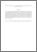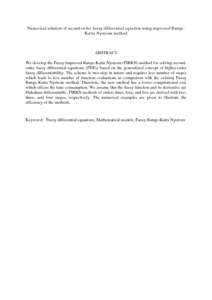# Numerical solution of second-order fuzzy differential equation using improved Runge-Kutta Nystrom method

## Citation

Rabiei, Faranak and Ismail, Fudziah and Ahmadian, Ali and Salahshour, Soheil (2013) Numerical solution of second-order fuzzy differential equation using improved Runge-Kutta Nystrom method. Mathematical Problems in Engineering, 2013. art. no. 803462. pp. 1-10. ISSN 1024-123X; ESSN: 1563-5147

## Abstract

We develop the Fuzzy Improved Runge-Kutta Nystrom (FIRKN) method for solving second-order fuzzy differential equations (FDEs) based on the generalized concept of higher-order fuzzy differentiability. The scheme is two-step in nature and requires less number of stages which leads to less number of function evaluations in comparison with the existing Fuzzy Runge-Kutta Nystrom method. Therefore, the new method has a lower computational cost which effects the time consumption. We assume that the fuzzy function and its derivative are Hukuhara differentiable. FIRKN methods of orders three, four, and five are derived with two, three, and four stages, respectively. The numerical examples are given to illustrate the efficiency of the methods.Preview
PDF (Abstract)
Numerical solution of second.pdfView Item